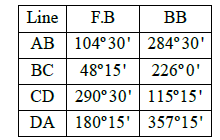Courses

# Test: Surveying- 3

## 25 Questions MCQ Test Civil Engineering SSC JE (Technical) | Test: Surveying- 3

Description
This mock test of Test: Surveying- 3 for Civil Engineering (CE) helps you for every Civil Engineering (CE) entrance exam. This contains 25 Multiple Choice Questions for Civil Engineering (CE) Test: Surveying- 3 (mcq) to study with solutions a complete question bank. The solved questions answers in this Test: Surveying- 3 quiz give you a good mix of easy questions and tough questions. Civil Engineering (CE) students definitely take this Test: Surveying- 3 exercise for a better result in the exam. You can find other Test: Surveying- 3 extra questions, long questions & short questions for Civil Engineering (CE) on EduRev as well by searching above.
QUESTION: 1

Solution:
QUESTION: 2

Solution:
QUESTION: 3

### Cross-staff is used for :

Solution:
QUESTION: 4

When 1cm on a map represents 10m on the ground, the representative fraction of the scale is

Solution:
QUESTION: 5

The distance between two brass rings in a surveyor's chain is

Solution:

Surveyor's chain or Gunter's chain is 66-foot (20.117 m) chain is divided into 100 links, usually marked off into groups of 10 by brass rings or tags which simplify intermediate measurement. Each link is thus 7.92 inches (20 cm) long.

QUESTION: 6

In a traverse survey, closing error means

Solution:
QUESTION: 7

The following bearing were observed while traversing with a compass, which stations are affected by local attraction ?Solution:
QUESTION: 8

A sewer is laid from a manhole A to a manhole B, 250 m away along a gradient of 1 in 125. If the reduced level of the invert at A is 205.75 m and the height of the boning rod is 3 m, the reduced level of the sight rail at B, is

Solution:
QUESTION: 9

The sum of the interior angles of a closed traverse is equal to :

Solution:
QUESTION: 10

The instrument used for accurate centering in plane table surveying is :

Solution:
QUESTION: 11

The best method of interpolation of contour is by

Solution:
QUESTION: 12

Levelling deals with measurement in

Solution:
QUESTION: 13

The correction in elevations due to the curvature and refraction is proportional to :

Where R is the radius of curvature of Earth and D is the horizontal distance.

Solution:
QUESTION: 14

Intersection method in plane table surveying is N most suitable for :

Solution:
QUESTION: 15

Mean Sea level (MSL) adopted by survey of India for reference is located at ?

Solution:
QUESTION: 16

Agate cap is fittted with a

Solution:
QUESTION: 17

Size of a theordolite is specified by

Solution:
QUESTION: 18

A telescope is said to be inverted if its

Solution:
QUESTION: 19

For which of the following permanent adjustments of theodolite, the spire test is used ?

Solution:
QUESTION: 20

A 'level line' is a

Solution:
QUESTION: 21

The following sights are taken on a 'turning point'

Solution:
QUESTION: 22

An ideal vertical curve to join two gradients, is

Solution:
QUESTION: 23

The rise and fall method

Solution:
QUESTION: 24

As applied to staff readings, the corrections for curvature and refraction are respectively

Solution:
QUESTION: 25

Hydrographic surveys deal with the mapping of

Solution: